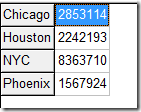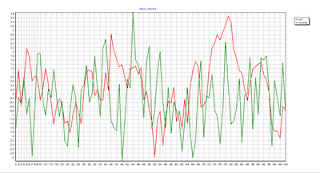## Saturday, August 29, 2009

### NLP modeling

Reading this presentation http://ice.uchicago.edu/2009_presentations/Skrainka_OptHessians.pdf I am missing one of the most valuable tools in NLP modeling: setting appropriate bounds. In my opinion (and experience) in NLP modeling there are three areas of attention when the model fails to yield an optimal solution:

1. Provide better initial solution
2. Do better scaling
3. Provide better bounds

Fixing these will help most ill-behaved models. These points are somewhat interrelated: a good bound may prevent the solver go into an area with scaling issues and a good bound also can change the initial point. Bounds are a very important tool in NLP modeling and should never be just ignored.

## Wednesday, August 26, 2009

In GAMS models I often see \$load being used to read data from a GDX file where \$loaddc is more appropriate. Here is why.

GAMS has the concept of domain checking (like type checking in programming languages). E.g. when we do

 GAMS File Data File set c 'cities' /    Chicago    Houston    NewYork    Phoenix /; parameter pop(c) 'population' / \$include population.inc /; Houston      2242193 Chicago      2853114 NYC          8363710 Phoenix      1567924

This fragment has a problem: the key for New York is not identical. So GAMS will issue an error message

11  NYC          8363710
****    \$170
**** LINE      3 INCLUDE     C:\projects\test\population.inc
**** LINE      8 INPUT       C:\projects\test\domain.gms
**** 170  Domain violation for element

Now we do the same with a GDX file, and \$load:

 GAMS File Data File set c 'cities' /     Chicago    Houston     NewYork    Phoenix  /; parameter pop(c) 'population'; \$gdxin population.gdx \$load pop display pop;When we run this fragment, we don’t see any error or warning! Of course the data is wrong: the population of New York is not read and is kept at its default value of zero. The display shows:

----     10 PARAMETER pop  population

Chicago 2853114.000,    Houston 2242193.000,    Phoenix 1567924.000

The \$load is just too unreliable to be used for anything that is larger than can be inspected by eye-balling. Compared to an old-fashioned include file it is really a step back.

A fixed version of \$load was introduced later and is called \$loaddc:

 GAMS File Data File set c 'cities' /     Chicago    Houston     NewYork    Phoenix  /; parameter pop(c) 'population'; \$gdxin population.gdx \$loaddc popThis will enforce domain checking:

**** 1 Domain errors for symbol pop
NYC
****            \$649

In general \$load’s should not be used. In some rare special cases a \$load can be used to select from a larger data set, but then you should add extra checks to prevent a situation as described here to slip through without error.

## Thursday, August 20, 2009

### GAMS: debugging tools

Earlier this week I was sitting next to an experienced GAMS modeler working together on a model, but I was nevertheless able to show him some tricks:
• Use \$stop to stop GAMS at a certain point and use GDX=X command line parameter to inspect the symbols as they are when stopped.
• The IDE gdx viewer is more powerful than many users know.
• If inside a loop, use
abort\$1 "stopped for debugging";
• If inside a loop and you want to stop at a certain iteration use something like:
abort\$(sameas(i,"iter10")) "stopped for debugging";
• Always simplify equations as much as possible by create intermediate sets and parameters. Equations are much more difficult to debug than sets or parameters.
• Loops are difficult to debug, not in the least because of an old issue in GAMS:
loop(i,
display i;
);

will display something else than expected: it will not show the current i but rather the complete set i. In addition when we use the abort\$1 trick we don't see at what point we stopped (I would like to see a loop-stack). Sometimes we need to introduce another singleton set -- a set with one element -- that keeps track of the current state of the loop set in order to create a simulated loop-stack.
Note: a bug in GAMS may introduce a symbol SameAs in the gdx file. This is largely a harmless bug.

## Sunday, August 16, 2009

### GAMS: allow declarations inside loop

Many newer languages allow for declarations almost anywhere. In a large GAMS model I had a case like this:

loop(i,
….much code here…
#include xxx.inc
….much code here…
);

Inside the include file I needed a local set so I could simplify some expressions. I can not place the set declaration there, but am forced to move it far, far away outside the loop. For small models this is not a big problem, but for very large models it would be beneficial to allow declarations inside loops so we can declare symbols close to where they are used.

## Wednesday, August 12, 2009

### Excel: many sheets

When you want to present a lot of data in Excel it may lead to a large number of sheets. In contrast to imposed limits on the number of rows and columns, Excel allows you to add an almost infinite number of sheets (only “limited by available memory”). However a large number of sheets can make it difficult to navigate for a user. The sheets are represented as tabs at the bottom of the window:

If there are more than a dozen or so it becomes cumbersome to find and select the correct tab.

Then in every sheet make sure it is easy to go back, e.g. by adding a link to the TOC page:

This now offers a completely alternative way of navigation in addition to using the sheet tabs. The above example spreadsheet is here.

## Friday, August 7, 2009

### GAMS: speed of loops

In GAMS models I often see loops where they are not really needed. E.g.

loop(i,
loop(j,
loop(k,
q(i,j,k) = 1;
)));

can be written as:

q(i,j,k) = 1;

Consider the larger fragment:

set i /i1*i200/;
alias(i,j,k);

parameter p(i,j,k);
p(i,j,k) = 1;

parameter q(i,j,k);
loop(i,
loop(j,
loop(k,
q(i,j,k) = 1;
)));

parameter q2(i,j,k);
loop((i,j,k),
q2(i,j,k) = 1;
);

The timing for the direct assignment to p(i,j,k) is 0.78 seconds. The time to populate q(i,j,k) is 18 seconds. The slightly different loop to fill q2 takes the same time: also 18 seconds. The difference is merely syntax. So if possible try not to use loops: not only is the code more compact, but it also performs better.

A more dramatic example is here (with card(i)=card(j)=card(k)=50):

 p(i,j,k) = max(0,p(i,j,k)); 0.031 secs loop((i,j,k),    if(p(i,j,k)<0, p(i,j,k)=0); ); 36.4 secs

## Tuesday, August 4, 2009

### GAMS: writing to a GDX file from different spots

For a large GAMS model I need to write to a GDX file from different places in the model. At compile time this can be done in some cases, e.g.:

\$gdxout x.gdx
… do stuff …

However compile-time gdx writing is only useful in exceptional cases. At execution time we are not allowed to append to a gdx file. The code:

… do stuff …

will cause x.gdx only to contain symbol b.

Unfortunately there is no good alternative for me:

• the model is too big and complex to save all data in extra parameters (so we can do a execute_unload once).
• the tool gdxmerge does not really merge unrelated gdx files very well: all symbols get an extra index which cause large problems in subsequent processing.
• writing a gams model that reads all these gdx files and then writes one gdx file is a lot of work, and would require a lot of memory if we call this from the main gams model.

### GAMS: \$onechoS

What is the difference between \$onecho and \$onechoS?

From the limited documentation it is not clear but \$onechoS is 100% identical to \$onecho. So no need to ever use \$onechoS: in my opinion (well actually experience) it will just cause confusion.

## Saturday, August 1, 2009

### Is this AR(1)?

We're looking to write a GAMS parameter that generates a series of random normal numbers with a user-chosen mean and user-chosen variance. But here's the twist: We need this generated series of numbers to have a user-chosen auto-correlation from one observation to the next. So if one number in the sequence exceeds the mean, we need the next one to be above it, too.
We're actually thinking of streamflows from one month or year to the next.  We know the flows' means and variances but also know their autocorrelation. Do you know of any kind of GAMS function that could generate this kind of parameter.  Possibly you could give us tips on how to write this function in GAMS?

I suspect you want to simulate an AR(1) process here. I.e.

X(t) = φ0 + φ1 X(t-1) + ε(t)

where ε(t) is normally distributed with mean 0 and variance σe2.

Now we have the following:

• Mean of x(t): μ = φ0/(1−φ1)
• Variance of x(t): σ2e2/(1−φ12)
• Autocorrelation coefficient: ρ=φ1

So given (μ,σ2,ρ) we can calculate

• φ1
• φ0= μ(1−φ1)
• σe22(1−φ12)

Now we can simulate X(t). In the hope that I did all the math correctly, we can now do in GAMS:

\$ontext

simulate ar(1) process

x(t) = phi0 + phi1*x(t-1) + N(0,esigma2)

mean of x(t): mu = phi0/(1-phi1)

variance of x(t):  var = esigma2/(1-phi1^2)

autocorrelation: rho = phi1

==>

given mu,var,rho we have

phi1 = rho

phi0 = mu*(1-phi1)

esigma2 = var*(1-phi1^2)
\$offtext

set
i0
'warmup-period' /i0-1*i0-100/
i
'number of observations to simulate' /i1*i50/
;

*
* these are the inputs
* mu = mean of x(t)
* s2 = variance of x(t)
* rho = autocorrelation coefficient
scalar
mu
'mean' /0/
s2
'variance' /2/
rho
'autocorrelation' /0.8/
;

scalars
phi0
phi1
esigma
;

phi1 = rho;
phi0 = mu*(1-phi1);
esigma = sqrt(s2*(1-sqr(phi1)));

*
* warm up
*
scalar x0 /0/;
loop(i0,
x0 = phi1*x0 + normal(phi0,esigma);
);

*
* simulate AR(1) process
*
parameter x(i);
loop(i,
x0 = phi1*x0 + normal(phi0,esigma);
x(i) = x0;
);

display x;

You may want to throw x(i) into a statistics package and see if it really behaves as you want.